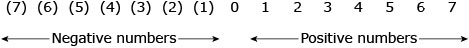Fundamentals of accounting

Start this free course now. Just create an account and sign in. Enrol and complete the course for a free statement of participation or digital badge if available.

Free course

# 2.8 Negative numbers and the use of brackets

Numbers smaller than zero (shown to the left of zero on the number line below in Figure 2) are called negative numbers. We indicate they are negative by enclosing them in brackets as shown in Figure 2.Figure 2 The number line

You may be used to seeing negative numbers indicated by the use of a minus sign ‘–’. However, because accountants conventionally use brackets so as to make it more obvious that a value is negative; this is the convention we adopt in this OpenLearn course. Negative numbers can be manipulated just like positive numbers and the calculator can deal with them with no difficulty as long as they are entered with a ‘–’sign in front of them.

## Box 3 Rules of negative numbers

The rules for using negative numbers can be summarised as follows:

Adding a negative number is the same as subtracting a positive

50 + (-30) = 50 – 30 = 20

Subtracting a negative number is the same as adding a positive

50 – (-30) = 50 + 30 = 80

Multiplication and division

A positive number multiplied by a negative gives a negative

20 x -4 = -80

A positive number divided by a negative gives a negative

20 / -4 = -5

A negative number multiplied by a negative gives a positive

-20 x -4 = 80

A negative number divided by a negative gives a positive

-20 / -4 = 5

Try to confirm the above rules for yourself by carrying out the following activity either manually or by means of a calculator.

## Activity 7 Use of negative numbers in maths operations

Calculate each of the following. (In this activity we will assume the convention that if a number is in brackets it means it is negative.)

1. (2) x (3)
To use this interactive functionality a free OU account is required. Sign in or register.
Interactive feature not available in single page view (see it in standard view).

6

1. 6 – (8)
To use this interactive functionality a free OU account is required. Sign in or register.
Interactive feature not available in single page view (see it in standard view).

14

1. 6 + (8)
To use this interactive functionality a free OU account is required. Sign in or register.
Interactive feature not available in single page view (see it in standard view).

(2)

1. 2 x (3)
To use this interactive functionality a free OU account is required. Sign in or register.
Interactive feature not available in single page view (see it in standard view).

(6)

1. (8) / 4
To use this interactive functionality a free OU account is required. Sign in or register.
Interactive feature not available in single page view (see it in standard view).

(2)

1. (8) / (4)
To use this interactive functionality a free OU account is required. Sign in or register.
Interactive feature not available in single page view (see it in standard view).

2

## Box 4 An important note about the use of brackets

Always remember that while a single number in brackets means that it is negative, the rule of BODMAS means that brackets around an ‘operation’ between two numbers, positive or negative, means that this is the first operation that should be done. The answer for a series of operations in an example such as 12 + (- 8 – 2) would thus be 2 according to the rules of BODMAS and negative numbers. It should be also noted that if 12 + (- 8 – 2) was given as 12 + ( (8) – 2) the answer would still be 2 as (8) is just another way of showing -8.